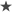Рейтинг:  0 / 5Sub Update()

Set CurrentWorkbook = Workbooks(ActiveWorkbook.Name)
Set InputWorksheet = CurrentWorkbook.Sheets("meBOM - OTH&HH")
Set OutputWorksheet = CurrentWorkbook.Sheets("1035159-VS")

Dim rng As Range, cell As Range

Dim N As Long
N = 1

Set rng = Range(Cells(3, 7), Cells(168, 7))

For Each cell In rng

If (cell.Value = "Yes") Then
N = N + 1
Range(Cells(N, 1), Cells(N, 6)).Copy
OutputWorksheet.Select
Range(Cells(N, 1), Cells(N, 6)).Select
OutputWorksheet.Paste

InputWorksheet.Select

End If
Next cell
End Sub